This text is meant to accompany class discussions. It is not everything there is to know about uniform circular motion. It is meant as a  prep for class. More detailed notes and examples are given in the class notes, presentations, and demonstrations (click here.)
Page
Description
1
Displacement and Constant Velocity
2

Modeling Motion with the Kinematics Formulas (Deriving the equations)

3
Solving Word Problems in Kinematics (Includes example video.)
4

g's ...Another Unit of Acceleration

5

"Drag Race Style" and Segmented Problems (Includes example videos of each.)

6 Kinematics and Free Fall Motion

 Objectives Students will be able to: 1 Describe the basic VECTOR motion concepts of; displacement, velocity, acceleration 2 Identify a number as being either displacement, velocity, acceleration, jerk or time based solely on its units. 3 List the values given in a word problem. These values will be listed and identified as either... initial position final position initial velocity final velocity average velocity acceleration time Also list the “implied” givens. (Implied from the context of the problem and/or the word choice such as the use “stop, drop, rest, and freefall.” 4 From memory, the following formulas will need to listed and used when solving word problems.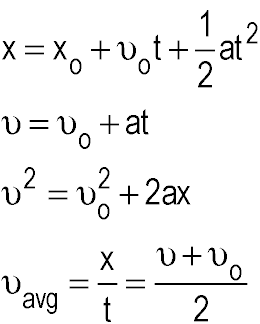5 List what the variables of xO, x, vO, v, vavg, a and t stand for 6 Write the proper S.I. units for the variables listed in the previous objective. 7 Solve word problems while demonstrating proper solution-communication techniques. This includes but is not limited to: List all the variables in a problem with units Show the formula(s) used to solve the problem with only variables Show the formula(s) used to solve the problem with numbers AND units Show any necessary math Show the answer with proper units 8 Solve word problems are involve multiple accelerations. (segmented problems). 9 Solve vertical free fall problems. 10 Be able to convert between accelerations in m/s2 and g’s. The test for this unit will be all problem solving. The Problems will be similar to the ones we've seen in class. The problems can be categorized into types, The simple problem that needs one equations to solve. The problem that is in freefall where the acceleration is "g" but the problem does not explicitly tell you a = 9.80 m/s2. A problem where you will need convert g"s into m/s2 in order to solve it. A "race style" problem. A "segmented" problem where you are given multiple accelerations in the description. Make sure you know how to solve a quadratic equation for the test. (You can use https://www.wolframalpha.com or the "PlySmlt2" on most TI-84 graphing calculators.)
Extra Resources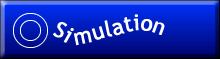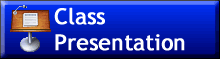Some notes Solve single unit conversion. Solve fractional unit conversion. Solve conversions where the units are raised to a power.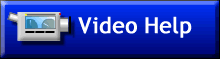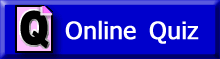Prob Link to see the video solution on YouTube 11 https://bit.ly/mwckinematics11 13 https://bit.ly/mwckinematics13 15 https://bit.ly/mwckinmatics15 17 https://bit.ly/mwckinematics17 19 https://bit.ly/mwckinematics 21a https://bit.ly/mwckinematics21a 21b http://bit.ly/mwckinematics21b 21c 21c 23 23 25 25 27 27 29 29 31 31a,b 31c 31cby Tony Wayne ...(If you are a teacher, please feel free to use these resources in your teaching.)

 The owner of this website does not collect cookies when the site is visited. However, this site uses and or embeds Adobe, Apple, GoDaddy, Google, and YouTube products. These companies collect cookies when their producs are used on my pages. Click here to go to them to find out more about how they use their cookies. If you do not agree with any of their policies then leave this site now.